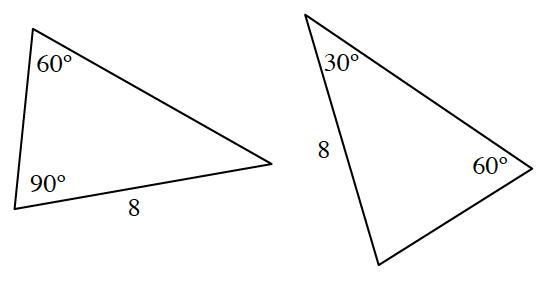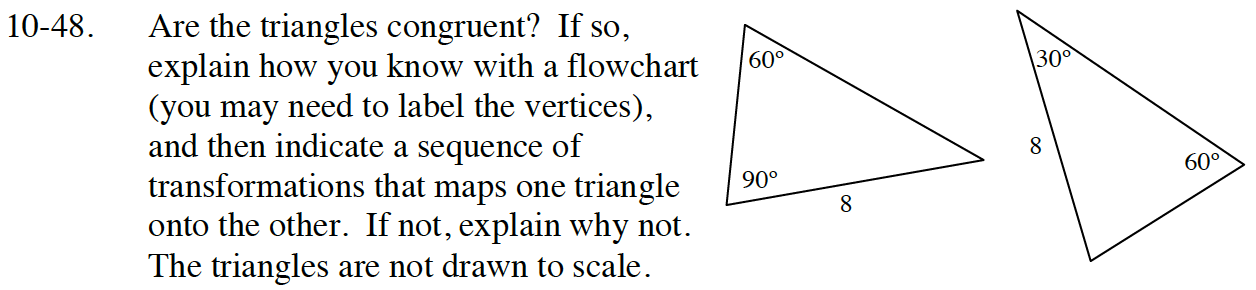### Home > CC4 > Chapter 10 > Lesson 10.1.3 > Problem10-48

10-48.

Are the triangles congruent? If so, explain how you know with a flowchart (you may need to label the vertices), and then indicate a sequence of transformations that maps one triangle onto the other. If not, explain why not. The triangles are not drawn to scale. Homework Help ✎Review the Math Notes box in section 7.1.7

Calculate the missing angle for each triangle.
Consider which triangle congruence condition could be met by these two triangles.